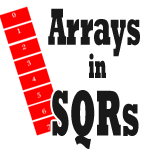# Arrays in SQRAn Array, like a database record, is organized as rows and columns. But unlike a database record, Arrays exists only in memory and NOT in the disk. One of the advantages that Arrays provide is that, once the data is populated into the array, it can be presented in multiple ways without further trips to the database there by making processing faster.

## Creating Arrays in SQR

Arrays must explicitly be declared before they can be used. This is how we create an array in SQR

```CREATE-ARRAY NAME=contracts SIZE=50 FIELD=contract_num:CHAR FIELD=contract_val:NUMBER```

The above code creates an array in SQR named contracts which has two fields which are contract_num and contract_val. Once the array is created, all numeric fields are defaulted to zero and everything else is set to NULL. So contract_num will have a value of NULL and contract_val will have zero.

Arrays are created at compile time. It is necessary that you provide the size of the array during this time. If you are not sure of how much data the array would need to hold, you have no option but to over allocate the size of the array so that the SQR program doesn’t fail.

## Using Arrays in SQR

Once we have the array created, we can place values into it and retrieve them for processing. The rows in arrays are numbered starting from zero. While using the array care needs to be taken to ensure that the limit specified while declaring the array are not crossed during operations.

The below section explains the different options available for array operations.

We can use the simple ‘Let’ statement to load values into an array. The ‘Put’ statement can also be use. Here’s how this can be done.

```LET contracts.contract_num(0) = 100000 PUT 100000 INTO contracts(0) contract_num PUT 100001 150000 INTO contracts(1)```

Line one and two does the same task of assigning the value 100000 to the contract_num field on the zeroth row of the array. Line 3 assigns the values 100001 to contact_num and 150000 to contract_val on the first row of the array contracts.

### Retrieving data from Arrays

In addition to the let command, SQR uses ‘Get’ command to retrieve values from the array. This is how it can be used.

```LET \$con_num = contracts.contract_num(0) GET \$con_num FROM contracts(0) contract_num GET \$con_num #con_val FROM contracts(1)```

The first two lines does the same task of copying the value of contract_num in the zeroth row to the \$con_num variable. The third line copies the value of contract_num into \$con_num and that of contract_val into con_val.

### Arithmetic Operations

The below arithmetic operations can be performed on arrays when all the variables are declared prior of these statements.

```ARRAY-ADD ARRAY-SUBTRACT ARRAY-MULTIPLY ARRAY-DIVIDE```

## Best Practices

These are some of the best practices are observed while using arrays in SQR.

1. Use #Define to set the size of the array. This way, understanding the size of the array would be easy and meaningful

```#DEFINE MAX_CONTRACTS 100 CREATE-ARRAY name = contracts size={MAX_CONTRACTS} FIELD = contract_num:CHAR FIELD = contract_val:NUMBER```

2. Reinitializing the array after use. This can be done by using the Clear-Array command

`CLEAR-ARRAY NAME = contracts`
##### 7 Responses
1.great posting to get the basic information on arrays quickly, Thank you very much.

•Happy to know that it was useful.

2.Pls change this contract_num will have a value of zero and contract_val will have NULL as contract_num will have NULL and contract_val will have a value of zero since contarct_num declared as character and contract_value declared as number

•Thanks for pointing this out, well spotted.

3.What is the maximum limit of an SQR array? Kindly advise. Thanks.

•In my tests, I specified a limit of 268402684 for the array in the example above and it worked.
However, when I specified a limit of 268402685, the program failed with the below error. I could not find any documentation supporting this though.

```Out of memory.
SQR for PeopleSoft: Program Aborting.
```

I’m on PeopleSoft/8.52/Windows 2008 R2

•Quote from PeopleBooks – ‘An SQR array can hold as many records as can fit in memory.’